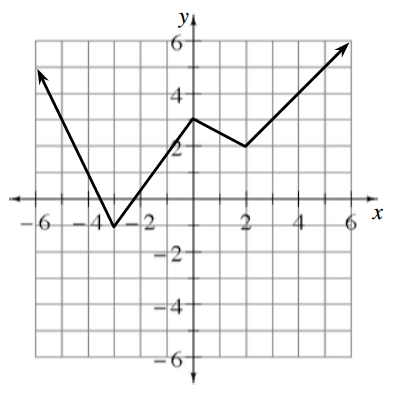### Home > PC3 > Chapter 2 > Lesson 2.1.3 > Problem2-34

2-34.

Given the graph of $y=f(x)$, sketch each of the following transformations.

1. $y=f(x-1)$

Shift the graph $1$ unit to the right.

1. $y=-f(x)$

Since $y=f(x)$, this transformation makes all of the $y$-values negative. How are positive and negative $y$-values related on a graph?